# Oscillatory Circuit

## oscillatory circuit

[′äs·ə·lə‚tȯr·ē ′sər·kət]
(electricity)
Circuit containing inductance or capacitance, or both, and resistance, connected so that a voltage impulse will produce an output current which periodically reverses or oscillates.
McGraw-Hill Dictionary of Scientific & Technical Terms, 6E, Copyright © 2003 by The McGraw-Hill Companies, Inc.
The following article is from The Great Soviet Encyclopedia (1979). It might be outdated or ideologically biased.

## Oscillatory Circuit

an electric circuit, containing an inductance coil and a capacitor, in which electric oscillations may be excited (Figure 1).

If at some moment of time the capacitor is charged up to a voltage V0 the energy concentrated in the electric field of the capacitor will be E0 = CV02/2, where C is the capacitance of the capacitor. If the capacitor is discharged, a current/will flow through the inductance coil and will increase until the capacitor is fully discharged. At this instant the electric energy of the oscillatory circuit Ec = 0, and the magnetic energy concentrated in the inductance coil EL = LI02/2, where L is the inductance of the coil and I0 is the maximum value of the current. There after the current through the coil begins to decrease and the voltage of the capacitor begins to increase in absolute magnitude but with opposite polarity. After a certain interval the current through the inductance will cease and the capacitor will be charged up to the voltage V0 the energy of the oscillatory circuit will again be concentrated in the charged capacitor. Then the process is repeated, but with a current of the opposite direction. The voltage at the capacitor plates varies according to the formula V= V0 cos ω0t, and the current in the coil varies according to the formula I = I0 sin ω0t; that is, natural harmonic oscillations of voltage and current with frequency ω0= 2π/T0, where T0 is the period of natural oscillations, are generated in the oscillatory circuit. In an oscillatory circuit the transfer of energy from the electric field of the capacitor to the magnetic field of the inductance coil, and vice versa, occurs twice during each period.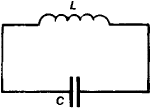Figure 1. An oscillatory circuit

However, in real oscillatory circuits some energy is lost. The energy is expended on heating the conductors of the coil, which have an effective resistance, and also on radiation of electromagnetic waves into the surrounding space or as a loss in dielectrics. Attenuation of oscillations occurs as a result of the losses. The amplitude of oscillations gradually decreases, so that the voltage at the capacitor plates now varies according to the formula V = V0eδt cos ωt where the coefficient δ = R/2L is the attenuation factor (or coefficient) and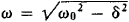is the frequency of the damped oscillations. Thus, the losses cause changes not only in the amplitude of oscillations but also in their period T = 2π/ω). The quality of an oscillatory circuit is usually described by its quality factor,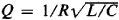. The magnitude of Q determines the number of oscillations completed by an oscillatory circuit after a single charging of its capacitor before the amplitude of oscillations decreases by a factor of e (e is the base of natural logarithms). If an AC generator with electromotive force (emf) U = U0 cos Ωt is connected to an oscillatory circuit (Figure 2), a complex oscillation that is the sum of natural oscillations of the circuit with frequency ω0 and forced oscillations with frequency Ω will arise in the circuit. At some time after the connection of the generator to the circuit, the natural oscillations of the circuit will decay and only the forced oscillations will remain. The amplitude of the stationary forced oscillations is determined by the equation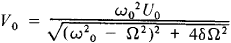that is, it depends not only on the amplitude of the external emf U0 but also on its frequency Ω. The relationship between the amplitude of oscillations in an oscillatory circuit and the frequency of the external emf is called the resonant characteristic of the circuit (Figure 3). A sharp increase in amplitude takes place at values of Ω close to the natural frequency ω0 of the oscillatory circuit. If Ω = ω0, Vmax exceeds the amplitude of the external emf U by a factor of Q. Since usually 10 < Q < 100, an oscillatory circuit makes possible the separation from a set of oscillations of those oscillations whose frequencies are close to ω0. It is precisely this property of oscillatory circuits (selectivity) that is used in practical applications. The frequency range (or band) ΔΩ near ω0 within which the amplitude of oscillations in an oscillatory circuit varies little (Figure 3) depends on the quality factor Q of the circuit, which is numerically equal to the ratio of the frequency ω0 of the natural oscillations to the frequency band width ΔΩ.Figure 2. Oscillatory circuit with a source of alternating emf U U0 cosΩt

To increase the selectivity of an oscillatory circuit it is necessary to increase Q. However, an increase of the quality factor is accompanied by an increase in the transition time required to establish steady-state oscillations in the circuit. In a circuit with a high quality factor changes in the amplitude of oscillation cannot keep up with the rapid changes in the amplitude of the external emf. In an oscillatory circuit the requirement for high selectivity is not compatible with the requirement for transmission of rapidly changing signals. Therefore, in some applications —for example, television signal amplifiers—the quality factor of the oscillatory circuit is lowered artificially. Schemes with two or more coupled oscillatory circuits are often used. With correctly chosen coupling, such systems exhibit an almost rectangular resonance curve (the dotted line in Figure 3).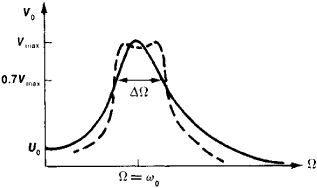Figure 3. Resonance curve of an oscillatory circuit: (ω0) frequency of natural oscillations, (Ω) frequency of forced oscillations, (ΔΩ) frequency band near ω0, on the boundaries of which the amplitude of the oscillations V = 0.7Vmax. The dotted line indicates the resonance curve of two coupled circuits.

In addition to the linear oscillatory circuits described above, with constant L and C, nonlinear oscillatory circuits are also used; in such circuits the parameters L and C depend on the amplitude of the oscillations. For example, if an iron core is inserted into an inductance coil, the magnetization intensity of the iron—and hence the inductance L of the coil—will vary with the variations of the current flowing through the coil. The oscillation period in such an oscillatory circuit depends on amplitude; therefore, the resonance curve acquires a slope and at large amplitudes becomes ambiguous (Figure 4). In the latter case amplitude jumps take place, even though the frequency Ω of the external emf changes smoothly. Nonlinear effects become more pronounced as the losses in an oscillatory circuit decrease. In oscillatory circuits with a low quality factor, nonlinearity does not affect the properties of the resonance curve.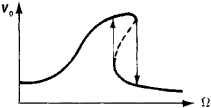Figure 4. Resonance curve of a nonlinear circuit

Oscillatory circuits are usually used as resonant systems for oscillators and amplifiers in the frequency range from 50 kilo-hertz to 250 megahertz. At higher frequencies sections of two-wire and coaxial lines, as well as cavity resonators, function as oscillatory circuits.

### REFERENCE

Strelkov, S. P. Vvedenie v teoriiu kolebanii. Moscow-Leningrad, 1951.

V. N. PARYGIN

References in periodicals archive ?
Determination of the resonance frequency [F.sub.off] of the oscillatory circuit with the MT OFF.
Determination of the frequency of the resonance Fair of the oscillatory circuit with the connected MT filled with air.
Determination of the frequency of the resonance of the [F.sub.nld] of the oscillatory circuit with connected MT filled with an anhydrous liquid nonpolar dielectric.
Four values of the resonant frequencies of the oscillatory circuit make it possible to determine four unknown quantities.
Calculations of the main circuit errors have shown that it possesses the properties of an oscillatory circuit. In the presence of failure of structural elements, oscillations appear in the main loop.
Together with a capacitor it forms an LCR oscillatory circuit (Fig.
A single-frequency example Foucault cardiograph, incorporating the sensor, operates at the resonance frequency of this oscillatory circuit. It consists of a sensor-based soft-regime LC-oscillator, which oscillates at the resonance frequency of the sensor, an amplitude detector and a low-frequency signal amplifier.
The sensor is expected to be a part of a self-oscillating device, which works in resonance with the sensor oscillatory circuit. Therefore it was natural to calculate the properties of the sensor just at its resonance frequency [f.sub.w] = 7.686 MHz at working conditions when it is loaded with a patient.
Therefore, oscillatory circuit frequency deviation expressed in terms of a constant surface of electrodes and a constant distance between them, electrical conductivity of water samples analyzed:
The signal conditioning circuit proposed in this work contains a variable frequency oscillator (Fig.6 (a)) with an inductive sensor in the oscillatory circuit, respiratory signal demodulator (b), band-pass filter (c) and amplifier (d).
The method based on recording oscillatory circuit parameters.
Rubezic, "Multiple STFT-based approach for chaos detection in oscillatory circuits," Signal Processing, vol.

Site: Follow: Share:
Open / Close